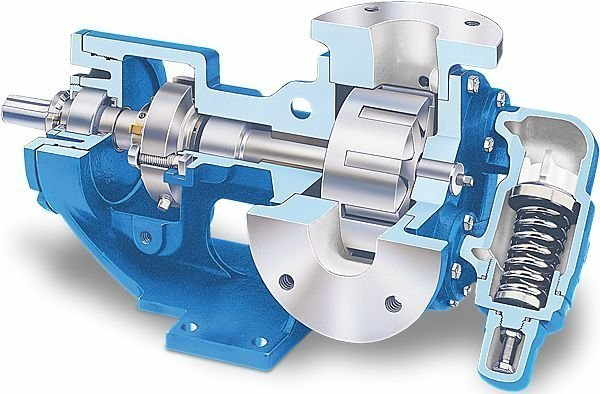# Design & Engineering support for modern electro-mechanical systems.

Hydrotech isn't just a distributor of quality industrial parts, We staff a full engineering department with experienced experts ready to design, assemble and integrate full system solutions.

# Hydraulic Technical Data### General Fluid Power Guidelines

Horsepower for driving a pump:

For every 1 hp of drive, the equivalent of 1 gpm @ 1500 psi can be produced.

Horsepower for idling a pump:

To idle a pump when it is unloaded will require about 5% of it's full rated power

Wattage for heating hydraulic oil:

Each watt will raise the temperature of 1 gallon of oil by 1° F. per hour.

Flow velocity in hydraulic lines:

Pump suction lines 2 to 4 feet per second, pressure lines up to 500 psi - 10 to 15 ft./sec., pressure lines 500 to 3000 psi - 15 to 20 ft./sec.; all oil lines in air-over-oil systems; 4 ft./sec.

## Basic Fluid Power Formulas

 Variable Word Formula w/ Units Simplified Formula Fluid Pressure - P (PSI) = Force (Pounds) / Area (Sq. In.) P = F / A Fluid Flow Rate - Q GPM = Flow (Gallons) / Unit Time (Minutes) Q = V / T Fluid Power in Horsepower - HP Horsepower = Pressure (PSIG) x Flow (GPM)/ 1714 HP = PQ / 1714

## Actuator Formulas

 Variable Word Formula w/ Units Simplified Formula Cylinder Area - A ( Sq. In.) = π × Radius (inch)2 A  =  π  × R2 (Sq. In.) = π × Diameter (inch)2 / 4 A  = π  × D2 / 4 Cylinder Force - F (Pounds) = Pressure (psi)   × Area (sq. in.) F  =  P × A Cylinder Speed - v (Feet / sec.) = (231 × Flow Rate (gpm)) / (12 × 60 × Area) v   =  (0.3208 × gpm) / A Cylinder Volume Capacity - V Volume = π × Radius2 × Stroke (In.) / 231 V = π × R2 × L / 231 (L = length of stroke) Cylinder Flow Rate - Q Volume = 12 × 60 × Velocity (Ft./Sec.) × Net Area(In.)2 / 231 Q = 3.11688 × v × A Fluid Motor Torque - T Torque (in. lbs.) = Pressure (psi) × disp. (in.3 / rev.) / 6.2822 T  =  P × d / 6.2822 Torque = HP × 63025 / RPM T = HP × 63025 / n Torque = Flow Rate (GPM) × Pressure × 36.77 / RPM T = 36.77 × Q × P / n Fluid Motor Speed - n Speed (RPM)  =  (231 × GPM) / Disp. (in.)3 n  =  (231 × GPM) / d Fluid Motor Horsepower - HP HP = Torque (in. lbs.) × rpm / 63025 HP  =  T × n / 63025

## Pump Formulas

 Variable Word Formula w/ Units Simplified Formula Pump Output Flow - GPM GPM = (Speed (rpm) × disp. (cu. in.)) / 231 GPM =  (n ×d) / 231 Pump Input Horsepower - HP HP =  GPM × Pressure (psi) / 1714 × Efficiency HP  =  (Q ×P) / 1714 × E Pump Efficiency - E Overall Efficiency = Output HP / Input HP EOverall = HPOut / HPIn X 100 Overall Efficiency = Volumetric Eff. × Mechanical Eff. EOverall = EffVol. × EffMech. Pump Volumetric Efficiency - E Volumetric Efficiency = Actual Flow Rate Output (GPM) / Theoretical Flow Rate Output (GPM) × 100 EffVol. = QAct. / QTheo. X 100 Pump Mechanical Efficiency - E Mechanical Efficiency = Theoretical Torque to Drive / Actual Torque to Drive × 100 EffMech = TTheo. / TAct. × 100 Pump Displacement - CIPR Displacement (In.3 / rev.) = Flow Rate (GPM) × 231 / Pump RPM CIPR = GPM × 231 / RPM Pump Torque - T Torque = Horsepower × 63025 / RPM T = 63025 × HP / RPM Torque = Pressure (PSIG) × Pump Displacement (CIPR) / 2π T = P × CIPR / 6.28

OUR PURPOSE

## Industry in Motion

American industry has never enjoyed more opportunity than it does today – or faced more challenges. Hydrotech is here to help you compete, evolve, lead and win with superior solutions in fluid power, automation, service & repair and connected technologies.